Magnetism

In ancient times Greece people found a rock that attracts iron, nickel and cobalt. They call them as “magnet “and magnetism comes from here. These rocks were used later by Chinese people to make compasses. Later scientists found that, magnets have always two poles different from electricity. Magnets have two ends or faces called “poles” where the magnetic effect is highest. In last unit we saw that there is again two polarities in electricity, “-“charges and “+” charges. Electricity can exist as monopole but magnetism exists always in dipoles North Pole (represented by N) and South Pole (represented by S). If you break the rock into pieces you get small magnets and each magnet also has two poles N and S.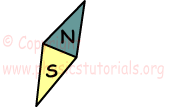Same poles of the magnet like in the electricity repel each other and opposite poles attract each other.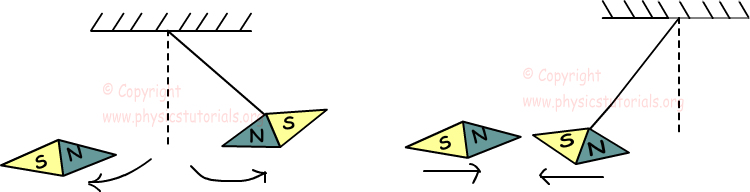Strengths of these forces depend on the distance between the poles and intensity of the poles.

Types of Magnets

In nature Fe3O4 is used as magnet. However, they can also produced by the people. They can have shapes rod, u shaped or horse shoe. Matters showing strong magnetic effect are called ferromagnetic; matters showing low magnetic effect are called diamagnetic and paramagnetic matters.

Coulomb’s Law for Magnetism

Effects of the two magnets to each other are inversely proportional to the square of distance between them and directly proportional to magnetic pole strenghts of each magnet. These forces are equal in magnitudes and opposite in directions.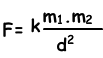F1=-F2

Where; k is the constant, m1 and m2 are the magnetic intensities of the poles and d is the distance between them.

Example: Find the forces exerted by the N poles of the magnets to each other.(k=10-7N.m2/(Amp.m)2.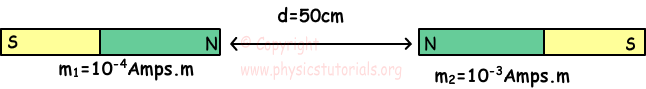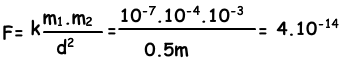Example: Two magnets are placed like given picture below. If the N pole  of the magnet exerts F force on the N poles of the second magnet, find the net force exerted on the first magnet.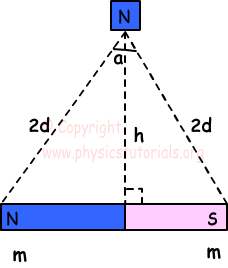We use the similarities of two triangle and get following equation.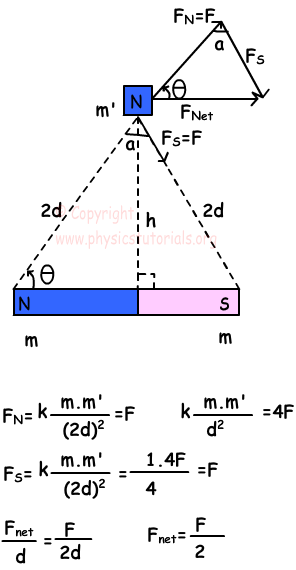Example: Three magnets are placed like given picture below. When the system released, magnet B gets closer to the magnet A. Find the possibilities of pole types of 1 and 4.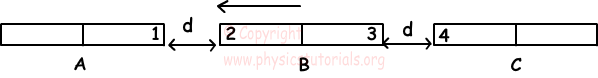If we assume that 1 is N pole, then since 1 attracts 2, 2 must be S, 3 is N and 4 is S.

If we assume that 1 is S pole, then since 1 attracts 2, 2 must be N, 3 is S and 4 is N.

Related